# Mapping in R

2015-07-31

Press "s" for presenter's notes
Code: mapping_in_r

## Purpose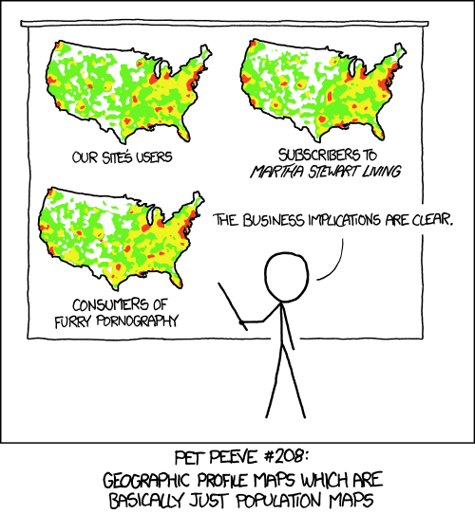### Map graphics communicate spatially distributed data

• Quick map plots help identify spatial dependencies (exploratory data analysis)
• Presenting results by administrative unit helps inform policy-making
• Tying data to relatable landmarks builds compelling narratives

You will leave with:

• High-level best practices for map graphics
• Tools to begin making map graphics using the R language
• Troubleshooting steps when you encounter problems

## Agenda

1. What makes a good map graphic?
2. Mapping in R
3. Troubleshooting mapping in R

## What makes a good map graphic?

Intuitive
Appropriate
Visually appealing

## Intuitive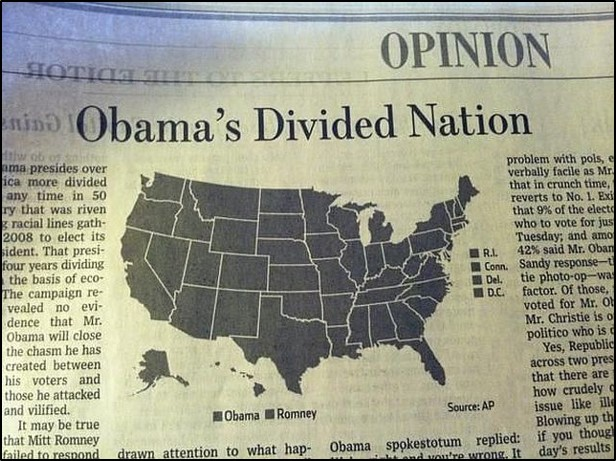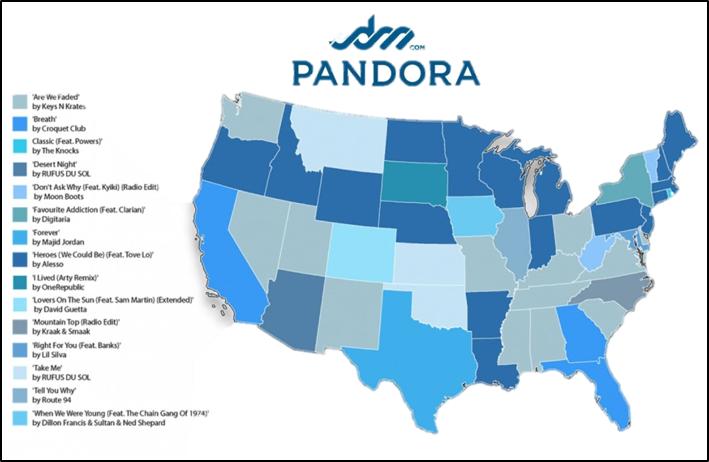## Appropriate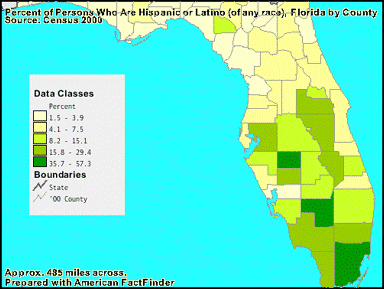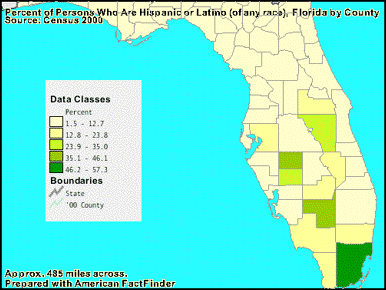## Visually Appealing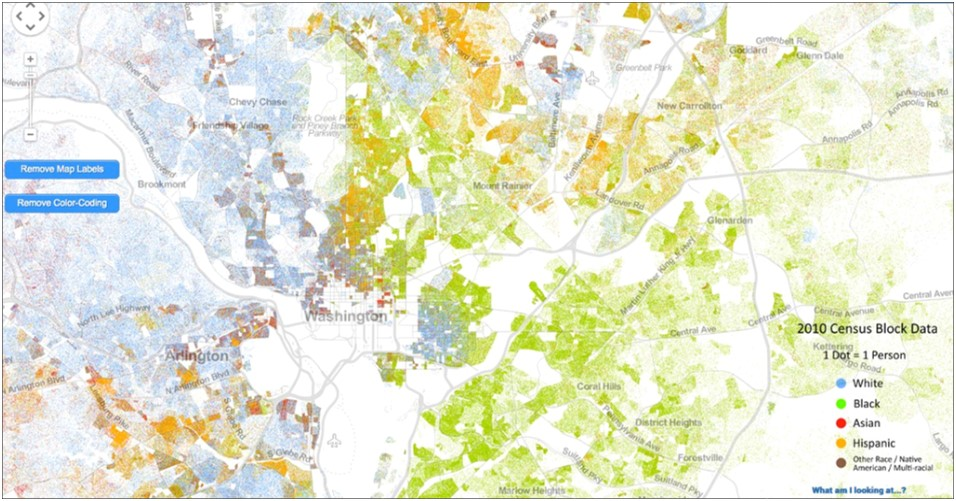## Mapping in R

Why R?
Raster and vector maps
Graphing over maps

## Why R?

• Free
• Community supported
• Attractive, informative, and fully reproducible graphics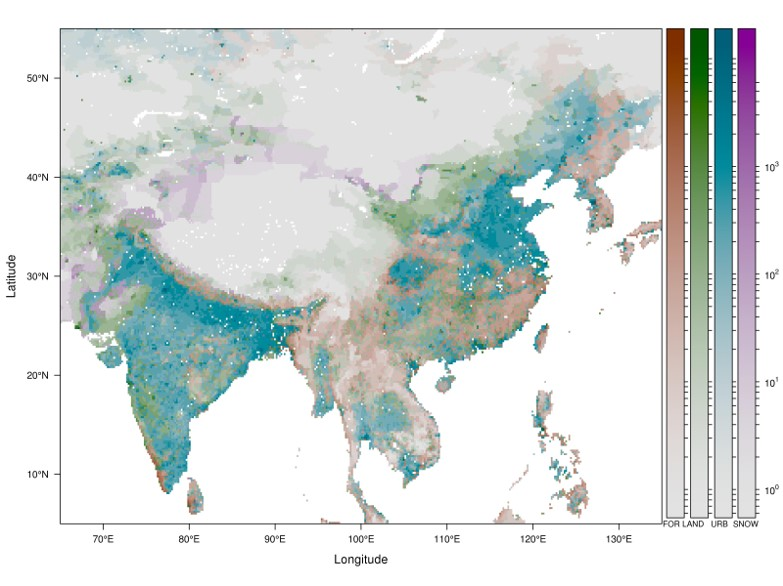## Why R.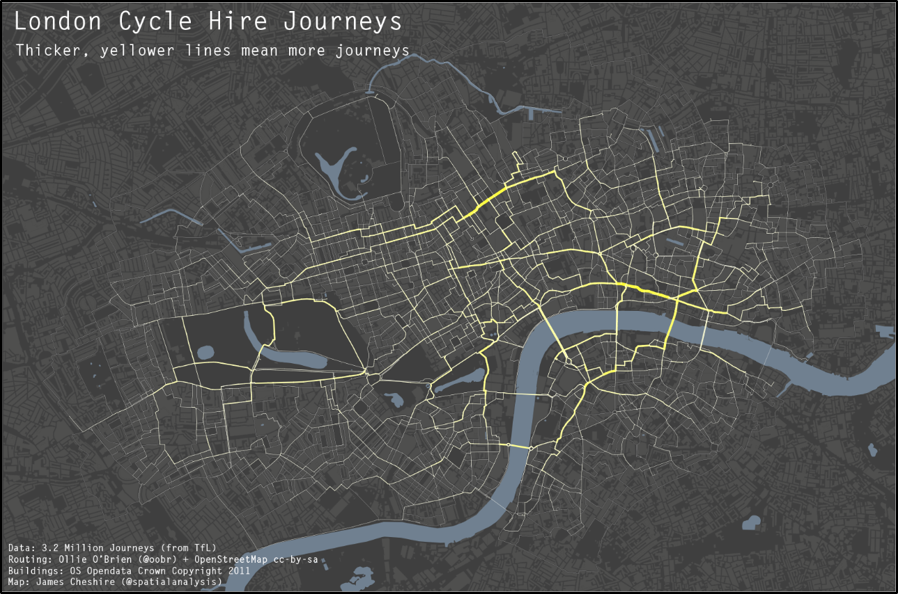qmap("601 New Jersey Ave NW, Washington, DC")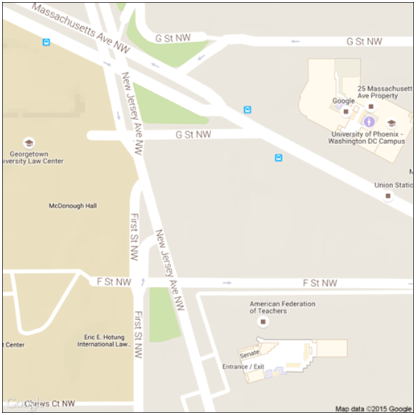eg <- data.frame(geocode(c("601 New Jersey Ave NW, Washington, DC",
"Union Station Metro, Washington, DC",
"Judiciary Square Metro, Washington, DC")))
qmplot(data = eg, x = lon, y = lat, zoom = 18, f = 1.1, size = I(3))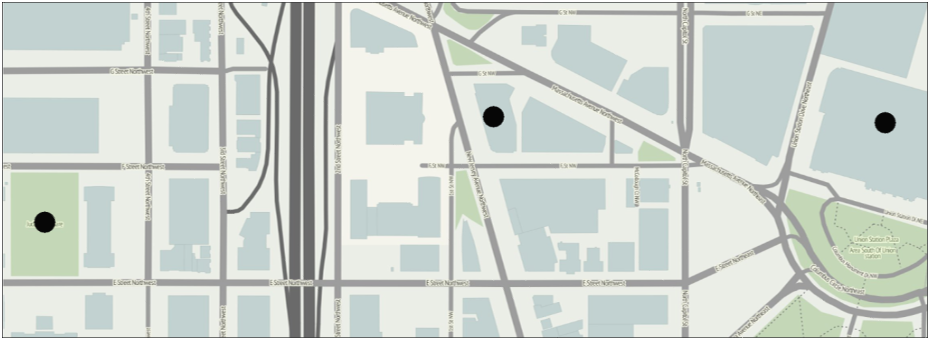## Rasters and Vectors

### Raster

• A raster map is an image of a map
• Appropriate for spatial point data
• More attractive, more default options, less customizable

### Vector

• A vector map is composed of polygons
• Appropriate for area-coded data
• Customizable, flexible applications, less attractive for equivalent work

## Raster maps from ggmap()


ggmap(get_map("601 New Jersey Ave NW, Washington, DC",
zoom = 12, source = "stamen", maptype = ...)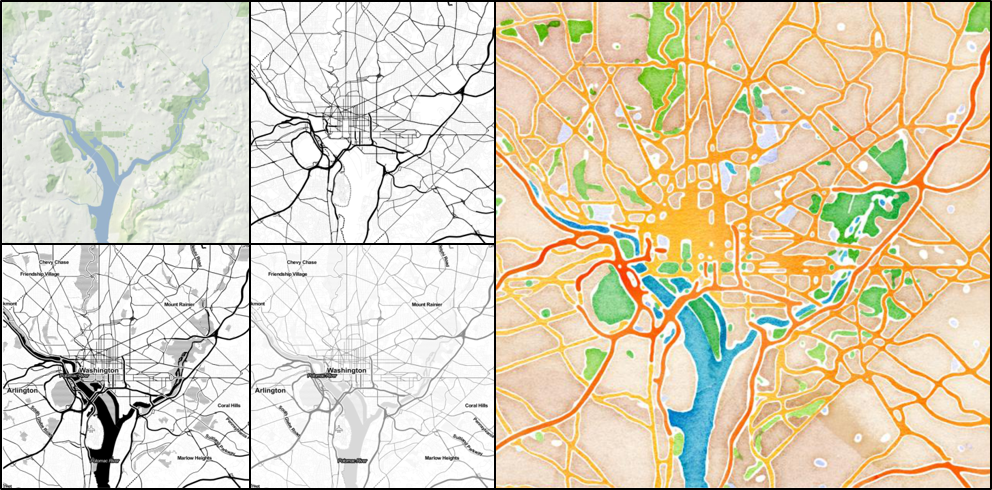## Vector maps from maps


map_data("world")
ggplot(world, aes(x = long, y = lat, group = group)) +
geom_polygon(aes(fill = region), color = "black")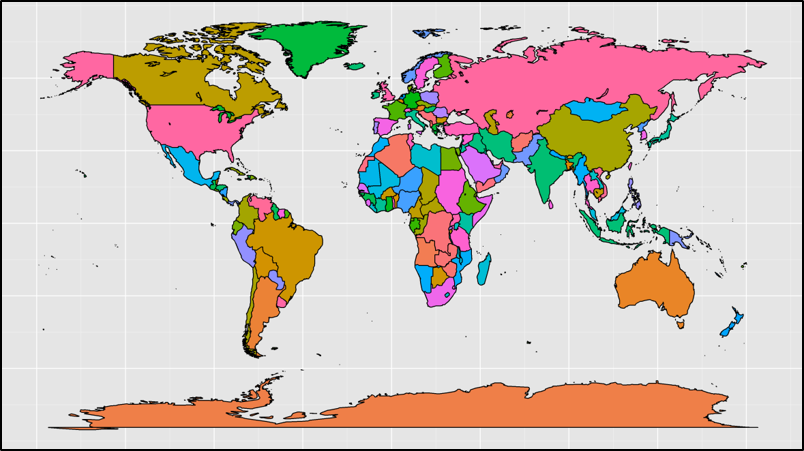## Vector maps from maps


map_data("world")
ggplot(world, aes(x = long, y = lat, group = group)) +
geom_polygon(aes(fill = region), color = "black") +
coord_map("ortho", orientation=c(41, -74, 0))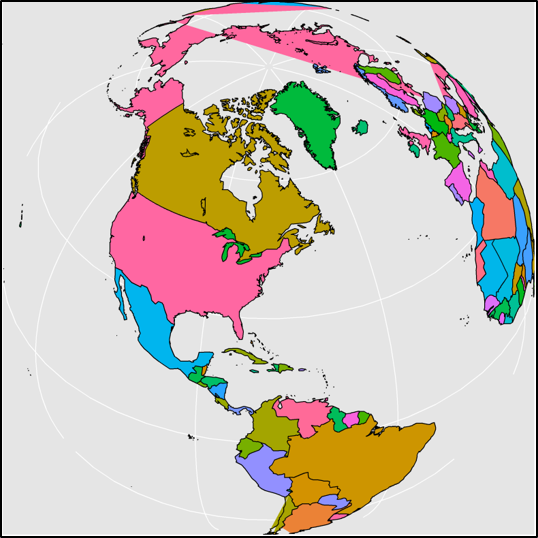## Vector maps from shapefiles


usa <- readOGR(dsn = "Inputs", "cb_2014_us_county_500k")
usa@data\$id = rownames(usa@data)
usa.points = fortify(usa, region = "id")
county = join(usa.points, usa@data, by = "id")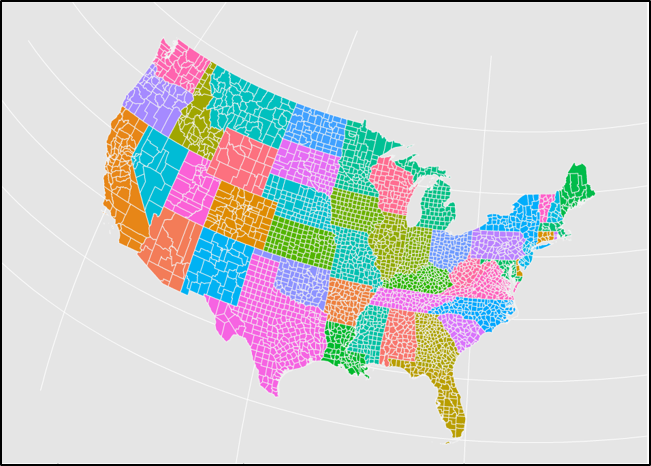## Mixing rasters and vectors


usa_raster <- get_map(bbox(county[,c("long", "lat")]),
maptype = "watercolor", zoom = 6)
ggmap(usa_raster, extent = "device",
base_layer = ggplot(aes(x = long, y = lat, group = group),
data = county)) +
geom_polygon(aes(fill = STATEFP, color = STATEFP), alpha = .3) +
coord_map(projection = "mercator")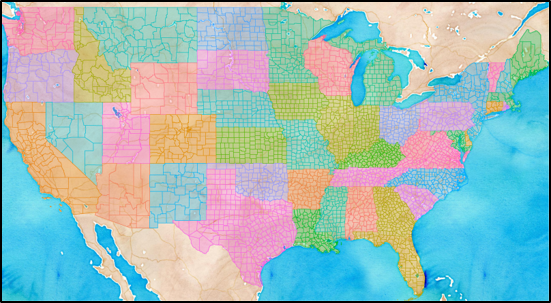## Graphing Data On Maps

The following slides are examples of four basic graphic map types:

• Dot density
• Choropleth
• Isopleth

The data used is the public HUD insured multifamily properties dataset and the US Census Quickfacts dataset.

## Dot density


ggplot(aes(x = long, y = lat), data = states) +
geom_polygon(aes(group = group), color = "grey95") +
geom_point(aes(x = LON, y = LAT), color = "#2db6e8", alpha = .6,
data = insured) +
coord_map()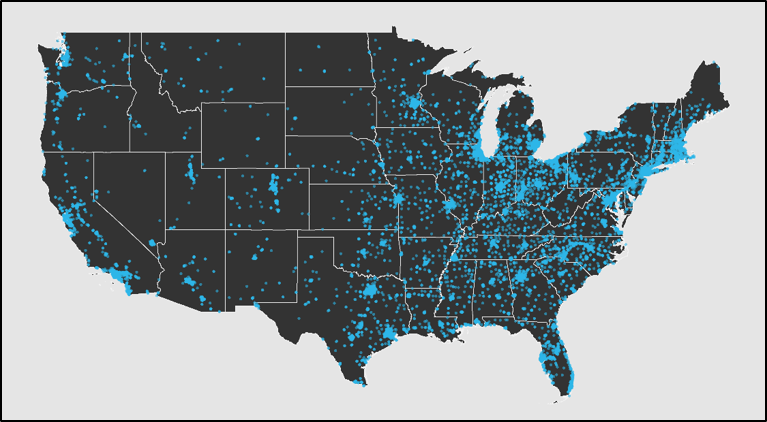ggplot(aes(x = long, y = lat), data = states) +
geom_polygon(aes(group = group), color = "grey95") +
geom_point(aes(x = LON, y = LAT, size = Unit_Total), color = "grey85",
data = cnty_count, shape = 21, fill = "#2db6e8") +
coord_map()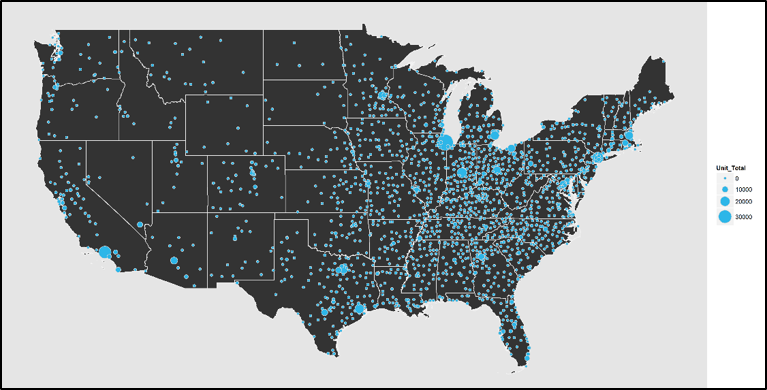## Choropleth


ggplot(aes(x = long, y = lat), data = county) +
geom_polygon(aes(group = group, fill = Trouble)) +
coord_map()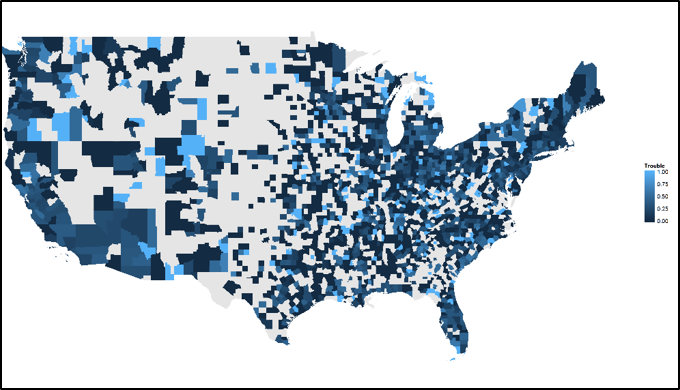## Isopleth


ggmap(dmv_map, base_layer =
ggplot(aes(x = LON, y = LAT, fill = CLIENT_GROUP_TYPE),
data = dmv_insured)) +
stat_density2d(aes(alpha = ..level..), bins = 3, geom = "polygon")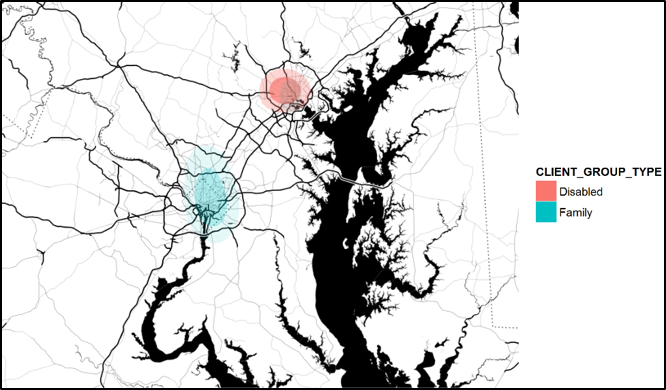## Troubleshooting

An example: JPS's problem
Local resources
Troubleshooting steps

## JPS's Problem

• Client presentation using geographic data
• Want to highlight relative performance across states
• Other mapping methods required proprietary software (SAS, ArcGIS), had limited customization (Excel), or required learning an entirely new open-source software on a short timeline (QGIS)

## JPS's Problem

What the data look like: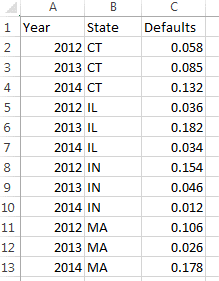How we want the data to look: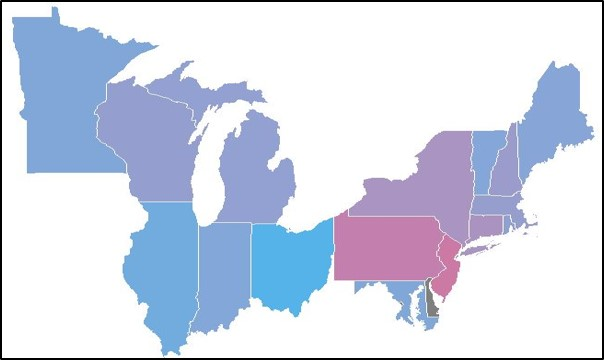## How the data looked after plotting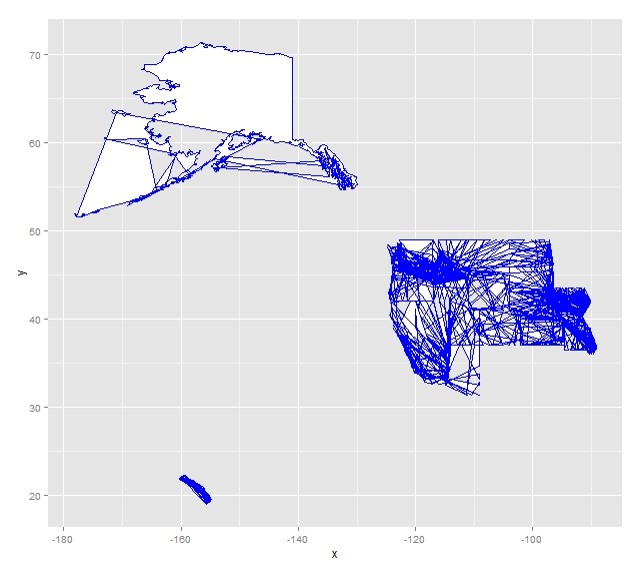## Troubleshooting

Typical sources of map graphic errors:

• Graphic generator methods (e.g., ggmap() not recognizing variables provided by the base layer)
• Data organization errors (e.g., ordering of vector points)
• Projection mismatching (e.g., vector in WGS84 and data points in NAT83)